# Vanishing Roots

##### Age 14 to 18

Published 2018

This resource is part of our Adventures with Complex Numbers collection

When you first learned about quadratic equations, you may have talked about equations with no real roots. No real roots...

Take a look at the video showing the graph of $y = x^2 - 6x +c$:

When $c>9$ the red dots disappear.
But what if those two red dots are still around somewhere?
Where could they be?
Is there a mathematical answer to that question that makes sense?

Let's think a little more about $y=x^2-6x+c$.
If we complete the square, we can write it as $y=(x-3)^2 - 9+c$.
The roots are the solutions to $(x-3)^2-9+c=0$,
which we can solve by rearranging to give $(x-3)^2=9-c$.

That's fine if $c \leq 9$, but if $c>9$ we would have to find the square root of a negative number, and we can't do that! Or can we?...

For a little more background on how our number system expands in order to solve a wider variety of equations, click below and read on...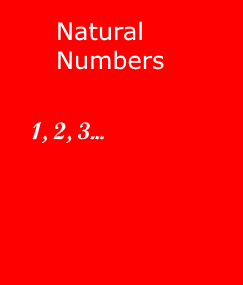Imagine a world where the only numbers are positive integers (whole numbers): 1, 2, 3, ...  We call this set the natural numbers, $\mathbb{N}$.

We can solve some equations involving positive integers, for example the equation $x^3 = 27$ has the solution $x=3$.

But we cannot solve every equation, even those where all the coefficients are positive integers.  For example, the equation $x+2 = 0$ has no solutions in the natural numbers.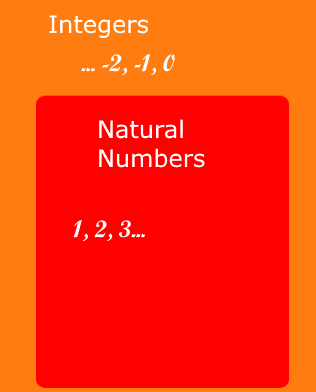So mathematicians extended the pool of numbers that we're allowed to use, by including zero and the negative integers.

The set of all integers (positive, negative and 0) is called $\mathbb{Z}$.  (Notice that every natural number is an integer.)

Now we can solve the equation $x+2 = 0$, and the equation $x^2 - 9 = 0$ has two solutions.

But again there are equations that we cannot solve, such as $2x - 3 = 0$.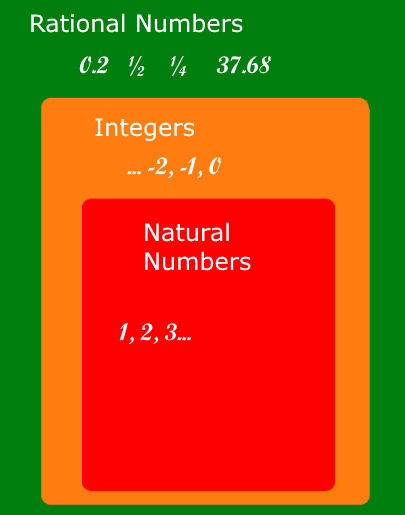So we extend the pool of numbers again, this time to give the set of rational numbers, called $\mathbb{Q}$.

These are the numbers of the form $\frac{x}{y}$ where $x$ and $y$ are integers and $y$ is not 0.  (Notice that every integer is a rational number.)

Now we can solve the equation $2x - 3 = 0$, but we still cannot solve some equations, such as $x^2 - 2 = 0$.  (This article includes a proof that $\sqrt{2}$ is irrational - it is not a rational number.)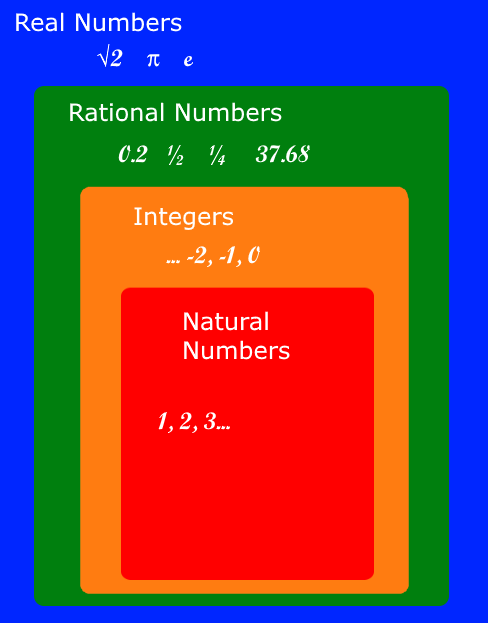We need the set of real numbers, called $\mathbb{R}$, which includes both the rational and irrational numbers.

We can now solve equations like $x^3 - 17 = 0$ and $x^2 - 5 = 0$.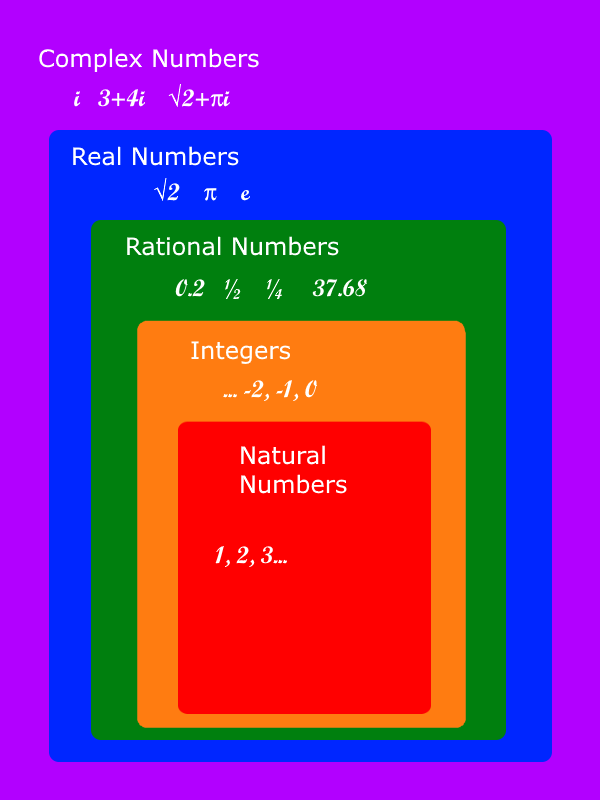But we still cannot solve the equation $x^2 + 2 = 0$ in the real numbers.  This is where the set of complex numbers, written $\mathbb{C}$, comes in.

We introduce a new number $i$, with the property that $i^2 = -1$, and then a complex number is one of the form $a + bi$ where $a$ and $b$ are real.

Now we can solve $x^2 + 2 = 0$.

Rather satisfyingly, we do not need to extend the complex numbers any further.  If we have any polynomial equation (such as a linear equation, quadratic equation, cubic equation,...) where all the coefficients are complex numbers, then we can always solve the equation in the complex numbers.  Better still, every quadratic with complex coefficients always has two complex roots, every cubic with complex coefficients always has three complex roots, and so on.  (Note that the roots might not necessarily be distinct: we can have repeated roots, where two or more roots are the same.)  This is known as the Fundamental Theorem of Algebra.

To explore Complex Numbers, the mathematical idea that explains where the roots go, try Opening the Door.

Notes and Background
This article gives some of the theoretical perspective about why complex numbers are important mathematically, but you may be interested to know that complex numbers are also of huge practical importance and are used in medicine, engineering, physics, technology, computing and many other fields. The article is part of the NRICH package Adventures with Complex Numbers, published in 2017 as part of a joint project between the University of Oxford and the University of Cambridge.